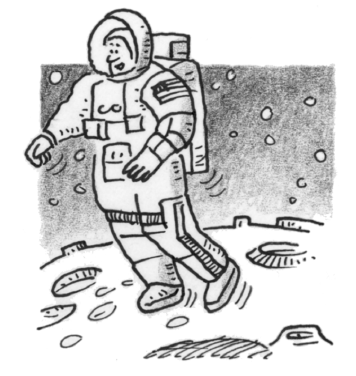### Home > APCALC > Chapter 6 > Lesson 6.1.4 > Problem6-48

6-48.

On the moon, the acceleration due to gravity is about $–5 \text{ ft/s}^2$. An astronaut jumps into the air with an initial upward velocity of $12$ ft/s.

1. How long is the astronaut off the ground?

$s(t)=\frac{1}{2}at^{2}+v_{0}t+s_{0}$

What do the zeros of $s\left(t\right)$ represent?

2. How far off the ground does the astronaut rise?

Find the $y$-value of the vertex of $s(t)$. You can use Algebra or Calculus.